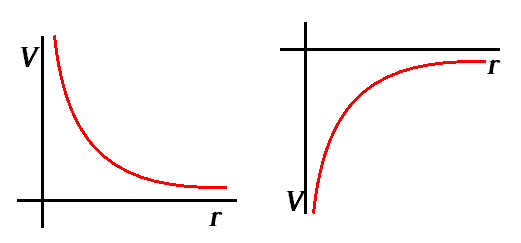# Help with understanding electric potential graphs

• Hannah7h

#### Hannah7hPlease could someone explain to me the graph on the left (for the positive source charge). I understand that the value for electric potential will be positive because of the source charge being positive, but why does it appear to decrease to zero at infinity, when the graph on the left (for the negative point charge) appears to increase to zero at infinity?

Thank you

The Coulomb potential of a static point charge in the origin is given by (using Heaviside-Lorentz units)
$$V(\vec{x})=\frac{q}{4 \pi |\vec{x}|}.$$
It's decreasing (increasing) for ##q>0## (##q<0##).

To remember why this is so, think about how the electric field is defined to begin with. It's given by the force on a very small test charge ##q'>0##. Now if the static charge that makes the Coulomb field is positive, the force is repulsive, i.e., the potential must be falling with distance such that it's negative gradient leads to a repelling force on a positive charge. For a negative charge it's repulsive and thus must be increasing. That in both cases the potential goes to 0 is just the choice of the zero-point of potential energy of the test charge which for convenience is chosen such that the potential energy (and thus the electric potential) goes to 0.

Indeed calculating the electric field gives
$$\vec{E}=-\vec{\nabla} V=\frac{q \vec{x}}{4 \pi |\vec{x}|^3},$$
pointing radially outward (away from the origin) when ##q>0## and radially inward (towards the origin) when ##q<0##. Accordingly the force on a positive test charge ##\vec{F}=q' \vec{E}## is repulsive for ##q>0## and attractive for ##q<0##, as it should be.

The Coulomb potential of a static point charge in the origin is given by (using Heaviside-Lorentz units)
$$V(\vec{x})=\frac{q}{4 \pi |\vec{x}|}.$$
It's decreasing (increasing) for ##q>0## (##q<0##).

To remember why this is so, think about how the electric field is defined to begin with. It's given by the force on a very small test charge ##q'>0##. Now if the static charge that makes the Coulomb field is positive, the force is repulsive, i.e., the potential must be falling with distance such that it's negative gradient leads to a repelling force on a positive charge. For a negative charge it's repulsive and thus must be increasing. That in both cases the potential goes to 0 is just the choice of the zero-point of potential energy of the test charge which for convenience is chosen such that the potential energy (and thus the electric potential) goes to 0.

Indeed calculating the electric field gives
$$\vec{E}=-\vec{\nabla} V=\frac{q \vec{x}}{4 \pi |\vec{x}|^3},$$
pointing radially outward (away from the origin) when ##q>0## and radially inward (towards the origin) when ##q<0##. Accordingly the force on a positive test charge ##\vec{F}=q' \vec{E}## is repulsive for ##q>0## and attractive for ##q<0##, as it should be.

THANK YOU SO MUCH I get it now